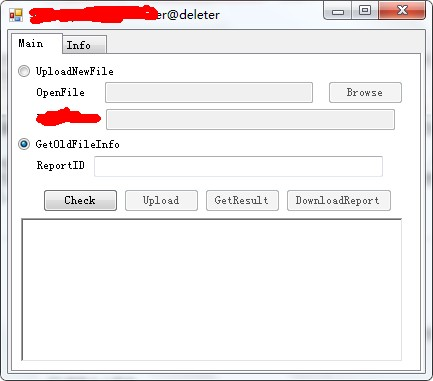# 开始

``````if(fileext!=".rar"&&fileext!=".rar"&&fileext!=""&&fileext!=".doc"&&fileext!=".xls"&&fileext!=".ppt"&&fileext!=".pdf")
{
return false;
}
``````

``````Random __gc* random = __gc new Random();
str = String::Concat(HttpContext::Current->Session[S"UserId"]->ToString(), random->Next(0x186a0, 0xf423f)->ToString(), str3);
``````

# 学术时间``````public static string ToGMTString(DateTime dt)
{
return dt.ToUniversalTime().ToString("r");
}

static void Main(string[] args)
{
for (int i = 0; i < 100000000; i++)
{
Console.Write(DateTime.Now.TimeOfDay.ToString());
Console.Write(" ");
Console.Write(ToGMTString(DateTime.Now));
Console.Write(" ");
Random r = new Random();
Console.WriteLine(r.Next(0x186a0, 0xf423f).ToString());
}
}
````````````public: Random();

public: Random(Int32 __gc* Seed);
``````

``````public: Random() : this(Environment::TickCount)
{
}
``````

``````public: __property static Int32 __gc* get_TickCount()
{
return Environment::nativeGetTickCount();
}
``````

`1000*60*60*60*24*30=155520000000=155.52G`

（只是猜想，未经测试，本人不负责）

# 后续